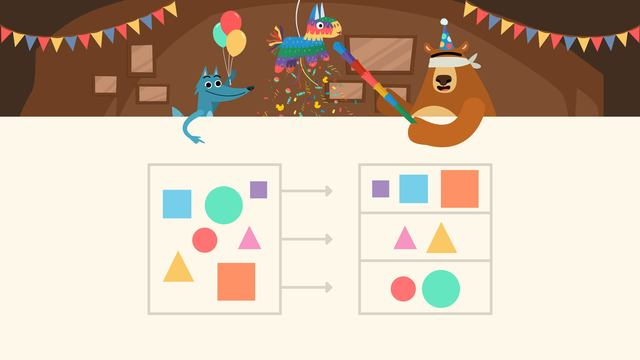# Collecting and Organizing Data

Content Collecting and Organizing DataRating

Ø 3.6 / 8 ratings
The authorsTeam Digital
Collecting and Organizing Data
CCSS.MATH.CONTENT.1.MD.C.4

## Collecting and Organizing Data

What is collecting and organizing data? Data is information we can collect and sort to help us organize and understand facts, numbers, and measurements. We can practice collecting data in two ways:

The first way is by observing, which means using our eyes to look at the data. The second way is to ask questions to collect more information about the data.## Collecting and Organizing Data – Example

Sometimes when we collect data there is a lot and it's hard to answer questions if the information is all mixed up. If we organize data into a chart, that can help answer questions more easily. For example, if we need to find out how many circles there are we first organize the shapes by putting them into categories.Now that we've organized the shapes into categories, we can look at the data with our eyes to find the answer. Even though we see circles, squares, and triangles, always remember what the question is asking: how many circles are there?

Shape Number
Squares 3
Triangles 3
Circles 3

If you look at the chart and count, you will see that there are three circles!

## Collecting and Organizing Data – Summary

Data is information we can collect and sort to help us understand facts, numbers, and measurements. Sometimes the data is mixed up so we organize it into categories to help us answer questions about the data.

Want some more collecting and organizing data practice? On this website there is a collecting and organizing data worksheet along with other activities, and exercises.

## Collecting and Organizing Data exercise

Would you like to apply the knowledge you’ve learned? You can review and practice it with the tasks for the video Collecting and Organizing Data.
• ### Highlight the data.

Hints

The food items are our data. We are organizing it using different colored highlighters.

Solution

Here we can see each food item highlighted to help us to organize our data.

• ### How many of each item are there?

Hints

Look at how many of each item is highlighted. Remember:

• tomatoes are highlighted in green.
• cheese is highlighted in blue.
• meatballs are highlighted in yellow.

We can see 6 tomatoes.

Solution
• There are 6 tomatoes.
• There are 3 meatballs.
• There are 5 pieces of cheese.
• ### Answer the questions using the data.

Hints

To find how many of each item there are you can read the number from the table.

To find out how many more tomatoes there are than pieces of cheese we need to subtract 5 (pieces of cheese) from 6 (tomatoes).

• 6 - 5 = ?

To find out how many fewer meatballs there are than pieces of cheese we need to subtract 3 (meatballs) from 5 (pieces of cheese).

• 5 - 3 = ?

To find out how many food items there are altogether we need to add each total.

• 6 + 3 + 5 = ?
Solution
1. How many tomatoes are there? 6
2. How many meatballs are there? 3
3. How many pieces of cheese are there? 5
4. How many more tomatoes than pieces of cheese are there? 1
5. How many fewer meatballs than pieces of cheese are there? 2
6. How many food items are there altogether? 14
• ### Have Nari and Gus organized their data correctly?

Hints

Count how many of each type of candy you can see.

Does the number you counted match Nari and Gus'? If not, highlight it.

Solution

Nari and Gus had miscounted a few of their data points.

• There were 7 gummy bears, not 8, so that was incorrect.
• There were 5 lollipops, so that was correct.
• There were 8 red sweets, not 7, so that was incorrect
• There were 4 yellow sweets, not 3, so that was incorrect.
• ### How many blue pieces of candy are there?

Hints

Remember, it is just the total of the blue pieces of candy that you are looking for here.

You are looking for all of the pieces of candy like this one, how many are there altogether?

Solution

There are 5 blue pieces of candy.

• ### Can you assign the data correctly?

Hints

Carefully count each item. Maybe you could create a table like this before you assign each item.

Once you know how many of each flower there are, you can assign it to the correct number.

Solution

Here are the totals of each flower.

• There were 8 tulips.
• There were 7 daffodils.
• There were 8 daisies.
• There were 3 roses.